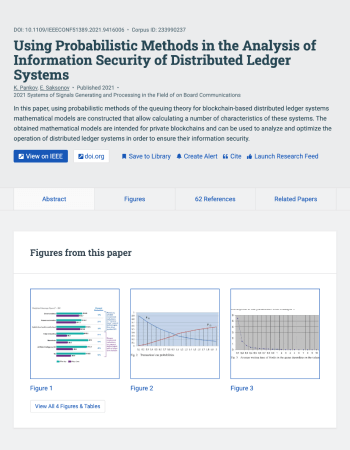Научная работа

# Pankov K. N., Saksonov E. A. Using Probabilistic Methods in the Analysis of Information Security of Distributed Ledger Systems, 2021.In this paper, using probabilistic methods of the queuing theory for blockchain-based distributed ledger systems mathematical models are constructed that allow calculating a number of characteristics of these systems. The obtained mathematical models are intended for private blockchains and can be used to analyze and optimize the operation of distributed ledger systems in order to ensure their information security.

МОСКОВСКИЙ ТЕХНИЧЕСКИЙ УНИВЕРСИТЕТ СВЯЗИ И ИНФОРМАТИКИ (МТУСИ)